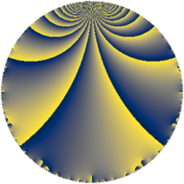# Properties

 Label 441.4.fLevel $441$ Weight $4$ Character orbit 441.f Rep. character $\chi_{441}(148,\cdot)$ Character field $\Q(\zeta_{3})$ Dimension $236$ Sturm bound $224$

# Related objects

## Defining parameters

 Level: $$N$$ $$=$$ $$441 = 3^{2} \cdot 7^{2}$$ Weight: $$k$$ $$=$$ $$4$$ Character orbit: $$[\chi]$$ $$=$$ 441.f (of order $$3$$ and degree $$2$$) Character conductor: $$\operatorname{cond}(\chi)$$ $$=$$ $$9$$ Character field: $$\Q(\zeta_{3})$$ Sturm bound: $$224$$

## Dimensions

The following table gives the dimensions of various subspaces of $$M_{4}(441, [\chi])$$.

Total New Old
Modular forms 352 256 96
Cusp forms 320 236 84
Eisenstein series 32 20 12

## Trace form

 $$236q - q^{2} + q^{3} - 451q^{4} + 7q^{5} - 19q^{6} + 18q^{8} - 43q^{9} + O(q^{10})$$ $$236q - q^{2} + q^{3} - 451q^{4} + 7q^{5} - 19q^{6} + 18q^{8} - 43q^{9} - 12q^{10} - 72q^{11} - 112q^{12} - 11q^{13} - 167q^{15} - 1639q^{16} + 30q^{17} + 310q^{18} - 26q^{19} + 14q^{20} - 3q^{22} - 11q^{23} + 465q^{24} - 2377q^{25} - 1336q^{26} - 272q^{27} - 285q^{29} - 498q^{30} + 79q^{31} - 823q^{32} + 412q^{33} - 279q^{34} - 373q^{36} + 244q^{37} + 455q^{38} - 703q^{39} + 84q^{40} + 458q^{41} - 260q^{43} + 1822q^{44} + 367q^{45} + 552q^{46} + 411q^{47} + 497q^{48} - 69q^{50} + 757q^{51} + 148q^{52} + 484q^{53} - 3133q^{54} - 462q^{55} + 1431q^{57} + 534q^{58} + 120q^{59} + 2330q^{60} + 439q^{61} - 2076q^{62} + 9506q^{64} - 1503q^{65} - 2176q^{66} - 398q^{67} + 1839q^{68} - 2091q^{69} - 24q^{71} - 1731q^{72} - 1574q^{73} - 2218q^{74} + 5933q^{75} + 667q^{76} + 3252q^{78} - 803q^{79} + 4424q^{80} + 4385q^{81} + 3606q^{82} + 2457q^{83} - 1068q^{85} - 2759q^{86} - 1763q^{87} - 483q^{88} - 4808q^{89} + 4172q^{90} + 3794q^{92} - 2381q^{93} - 906q^{94} - 3782q^{95} - 800q^{96} - 1262q^{97} - 8197q^{99} + O(q^{100})$$

## Decomposition of $$S_{4}^{\mathrm{new}}(441, [\chi])$$ into newform subspaces

The newforms in this space have not yet been added to the LMFDB.

## Decomposition of $$S_{4}^{\mathrm{old}}(441, [\chi])$$ into lower level spaces

$$S_{4}^{\mathrm{old}}(441, [\chi]) \cong$$ $$S_{4}^{\mathrm{new}}(9, [\chi])$$$$^{\oplus 3}$$$$\oplus$$$$S_{4}^{\mathrm{new}}(63, [\chi])$$$$^{\oplus 2}$$